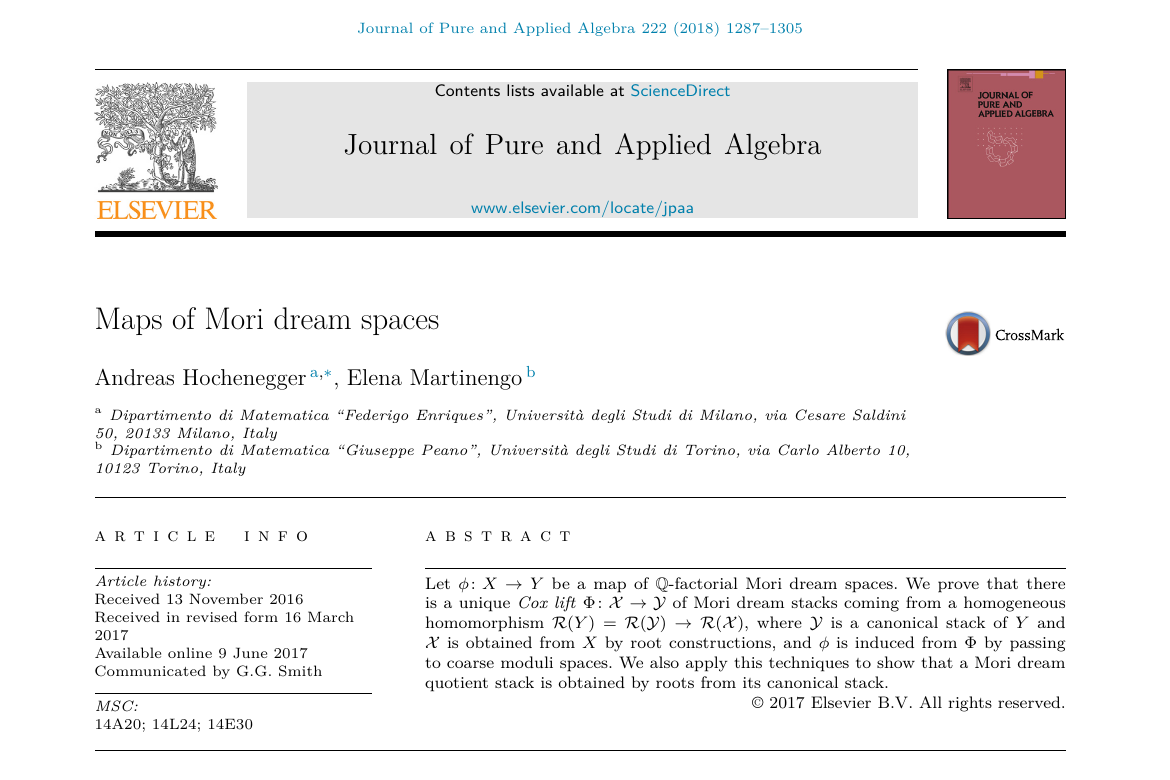### Maps of Mori Dream Spaces

##### Journal of Pure and Applied Algebra, 222(6):1287-1305, 2018.

Parole chiave

Abstract. Let $\phi: X \to Y$ be a map of $\mathbb{Q}$-factorial Mori dream spaces. We prove that there is a unique Cox lift $\Phi: \mathcal{X} \to \mathcal{Y}$ of Mori dream stacks coming from a homogeneous homomorphism $\mathcal{R}(Y) = \mathcal{R}(\mathcal{Y}) \to \mathcal{R}(\mathcal{X})$, where $\mathcal{Y}$ is a canonical stack of $Y$ and $\mathcal{X}$ is obtained from $X$ by root constructions, and $\phi$ is induced from $\Phi$ by passing to coarse moduli spaces. We also apply this techniques to show that a Mori dream quotient stack is obtained by roots from its canonical stack.

© 2021 GAA@polimi Generato con Hugo e inspirato al tema Resume Ultimo aggiornamento: 8 Giugno 2022